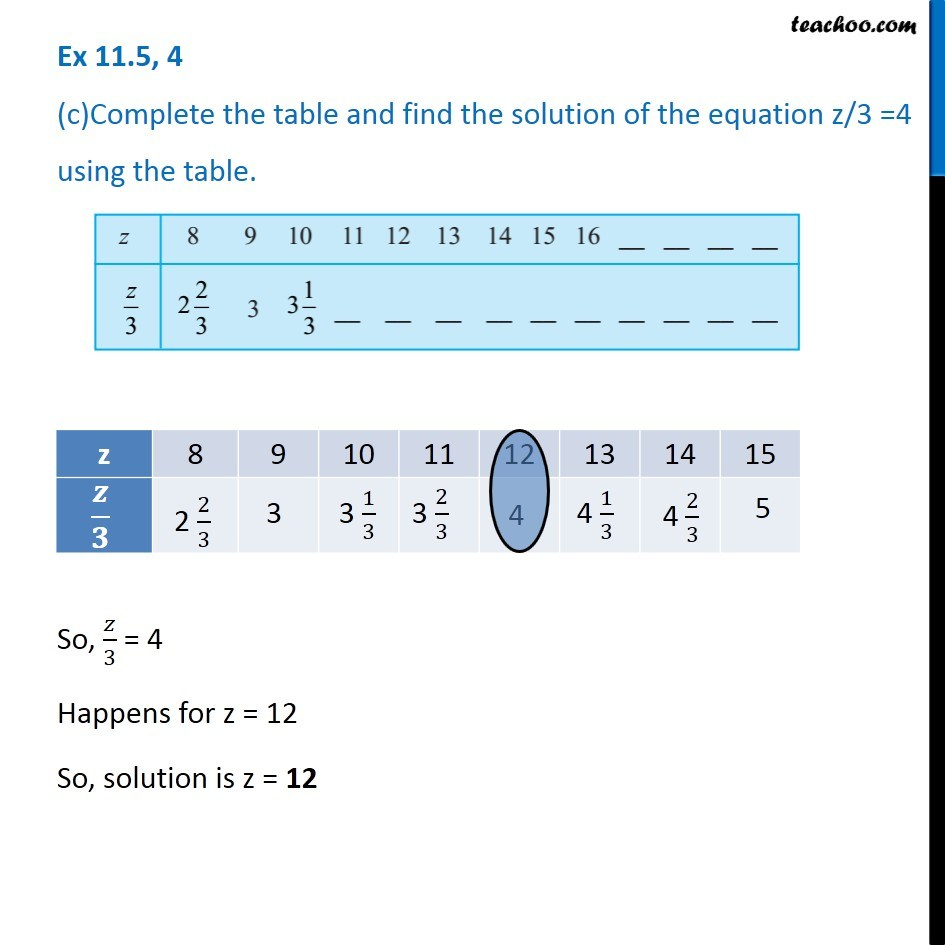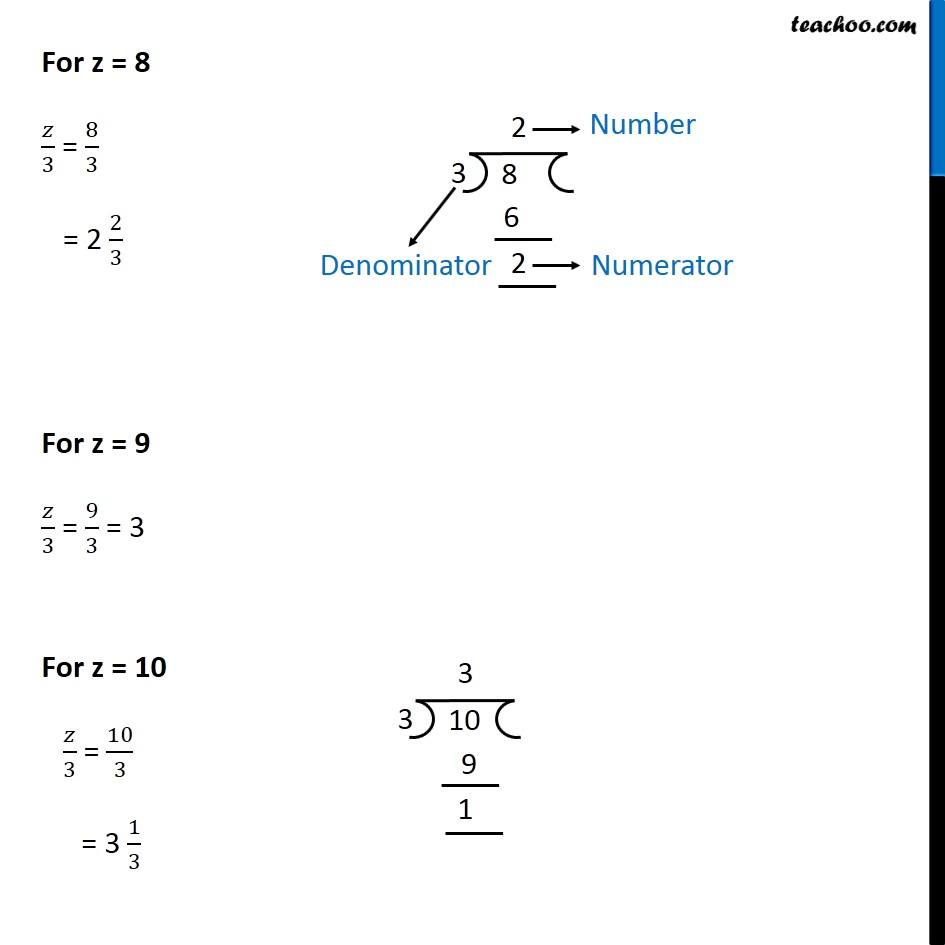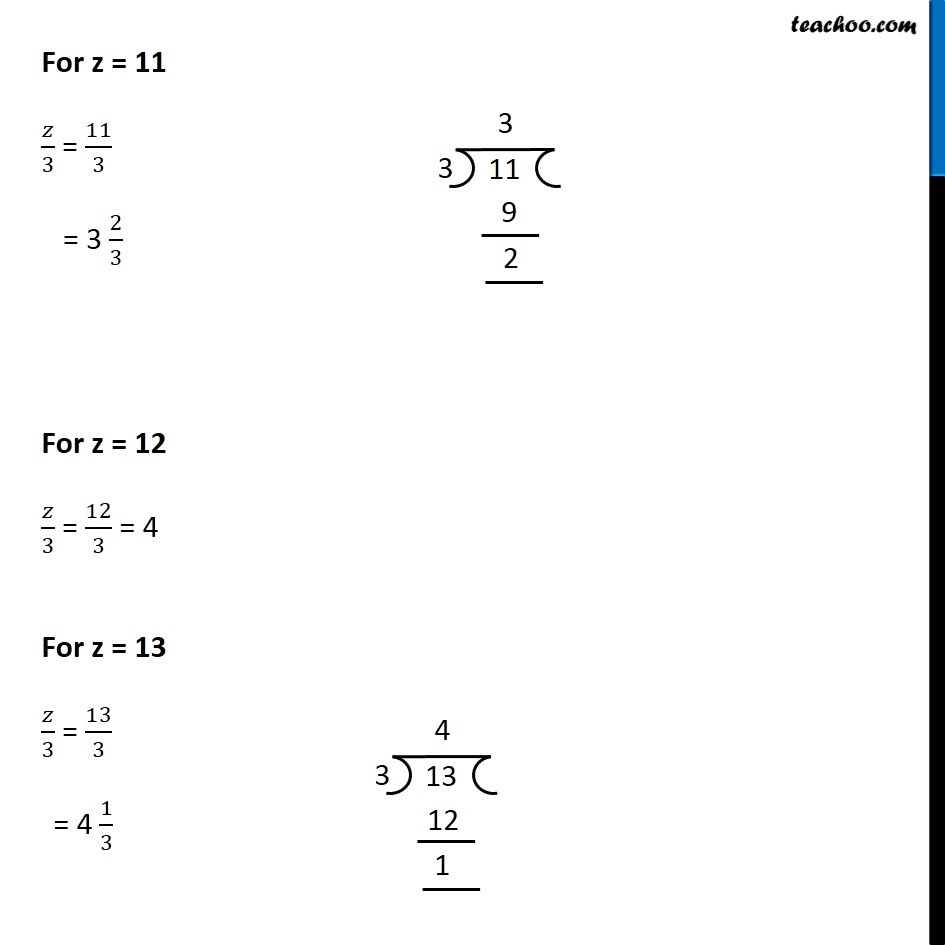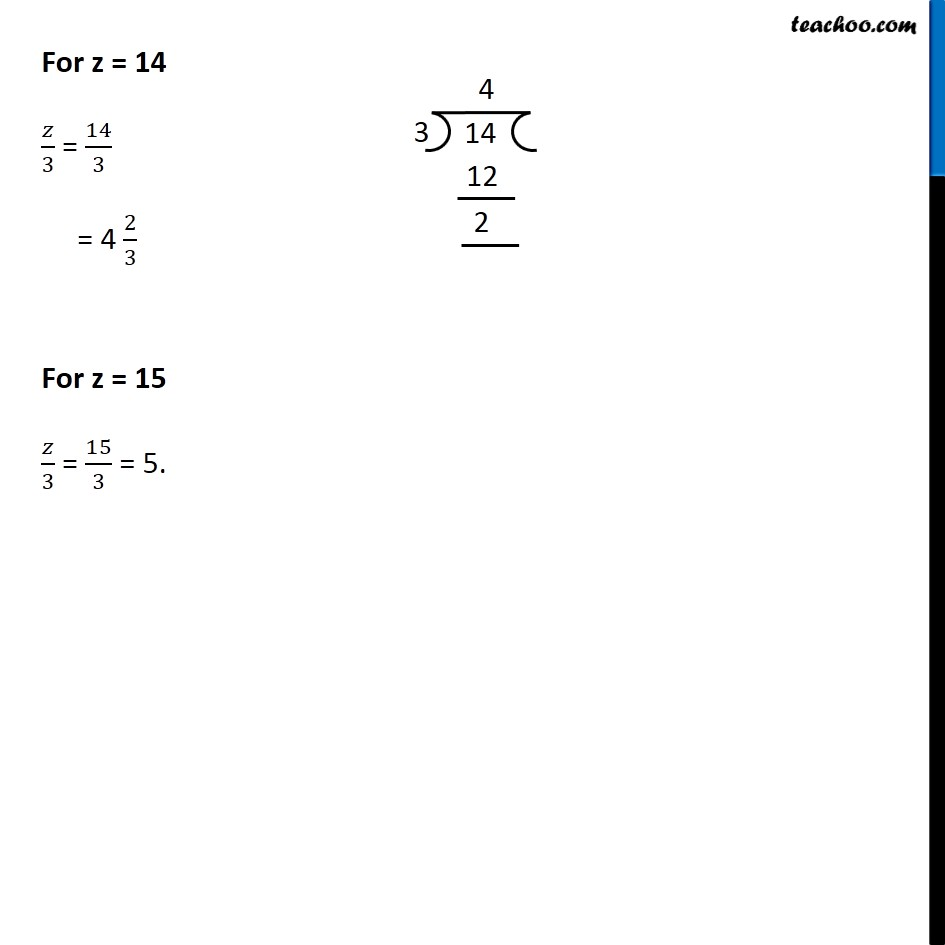Finding solutions of Algebra Equations - WorksheetLearn in your speed, with individual attention - Teachoo Maths 1-on-1 Class

### Transcript

Question 4 (c)Complete the table and find the solution of the equation z/3 =4 using the table.So, 𝑧/3 = 4 Happens for z = 12 So, solution is z = 12 For z = 8 𝑧/3 = 8/3 = 2 2/3 For z = 9 𝑧/3 = 9/3 = 3 For z = 10 𝑧/3 = 10/3 = 3 1/3For z = 11 𝑧/3 = 11/3 = 3 2/3 For z = 12 𝑧/3 = 12/3 = 4 For z = 13 𝑧/3 = 13/3 = 4 1/3For z = 14 𝑧/3 = 14/3 = 4 2/3 For z = 15 𝑧/3 = 15/3 = 5.